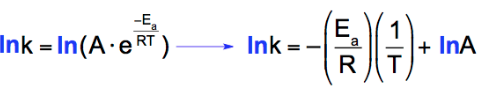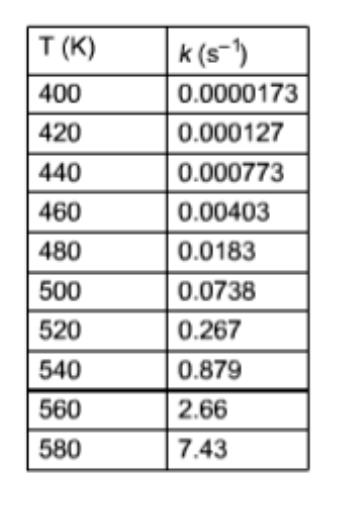# Problem: The rate of a certain reaction was studied at various temperatures. The table shows temperature (T) and rate constant (k) data collected during the experiments. Plot the data, and then answer the following questions. What is value of the activation energy, E a (kJ•mol -1), for this reaction? What is the value of the pre-exponential factor (sometimes frequency factor), A (s -1), for this reaction?

###### FREE Expert Solution
90% (378 ratings)
###### FREE Expert Solution

To plot the data we need to use the Plot-wise approach of the Arrhenius Equation. The Arrhenius equation can be written in a non-exponential form or straight-line form.90% (378 ratings)###### Problem Details

The rate of a certain reaction was studied at various temperatures. The table shows temperature (T) and rate constant (k) data collected during the experiments. Plot the data, and then answer the following questions.

What is value of the activation energy, a (kJ•mol -1), for this reaction?

What is the value of the pre-exponential factor (sometimes frequency factor), A (s -1), for this reaction?What scientific concept do you need to know in order to solve this problem?

Our tutors have indicated that to solve this problem you will need to apply the Arrhenius Equation concept. You can view video lessons to learn Arrhenius Equation. Or if you need more Arrhenius Equation practice, you can also practice Arrhenius Equation practice problems.

What is the difficulty of this problem?

Our tutors rated the difficulty ofThe rate of a certain reaction was studied at various temper...as high difficulty.

How long does this problem take to solve?

Our expert Chemistry tutor, Dasha took 11 minutes and 25 seconds to solve this problem. You can follow their steps in the video explanation above.

What professor is this problem relevant for?

Based on our data, we think this problem is relevant for Professor Peters' class at UK.